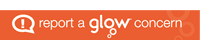# SNSA Numeracy Bands Explained

Where pupils have sat the S3 SNSA Numeracy Assessment, the following bands show the overall capacity demonstrated:

Band 12 and Above

Learners in this band are typically able to apply the correct operations to complete calculations involving 3-digit numbers, fractions and ratios. They are typically able to solve speed distance time problems involving conversion of units of time and length. Learners can also interpret two-way tables and Venn diagrams to find the probabilities of events and complementary events.

Band 11

Learners in this band can typically interpret problems and apply the correct operations to complete calculations. They can interpret rates and proportions to solve problems and can find areas and perimeters of shapes using limited given information. Learners can interpret two way tables, segmented column graphs and line graphs and calculate probabilities of complementary events and express these as decimal fractions.

Band 10

Learners in this band are typically able to perform calculations involving negative numbers and the subtraction and multiplication of decimal fractions. They can solve word problems involving proportion. Learners are able to solve problems involving duration, conversion of units of time and the unitary method. They can interpret pie charts and two-column graphs to find unknown values.

Band 9

Learners in this band can typically round whole numbers and decimal fractions to given accuracy levels and compare and order fractions with different denominators. They can calculate volumes of cuboids and compare masses to 3-decimal places. They can typically match pie charts and tables showing the same information, and can calculate the expected value given the percentage probability of an event.

Band 8

Learners in this band are typically able to identify an appropriate number sentence to represent a problem. They can calculate durations across hours, minutes and seconds, and calculate the probability of an event, expressing it as a decimal fraction. They can also identify the most appropriate sample selection for a given survey question.

Band 7 and Below

Learners in this band are typically able to solve single-step word problems involving any of the four operations. They can convert between 24- and 12- hour time. They can also use interpret a pictograph that uses symbols and part symbols to represent multiple units. They can interpret statements describing probabilities and match these to probabilities expressed as percentages.

Where pupils have sat the P7 SNSA Numeracy Assessment, the following bands show the overall capacity demonstrated:

Band 11 and Above

Learners in this band can typically interpret problems and apply the correct operations to complete calculations involving estimation, the multiplication of 2-digit numbers . They can solve problems involving a fraction of a fraction and can calculate a percentage of a given value. They can solve problems involving the areas of rectangles and convert units of mass.

Band 10

Learners in this band can typically solve word problems involving proportional reasoning to find fractions of a group and can also add fractions and decimal fractions. They can calculate durations and convert between units, e.g. for length or volume, and read scales for values between labelled marks. They can interpret pie charts and determine the probability of outcomes on a simple spinner.

Band 9

Learners in this band can typically apply all four operations to solve problems involving whole numbers up to 1 000 000 and with money. They can order fractions and decimal fractions. They can convert time in fractions of an hour to minutes, use coins to show change from £20 or £30. They can interpret Venn diagrams with three overlapping sets of data.

Band 8

Learners in this band are typically able to solve a multi-step word problem, use place value in numbers with up to 6 digits and identify and order 3-digit numbers represented in words. They can calculate durations across hours, minutes and seconds within a day and identify the group that makes up a population for a particular kind of survey.

Band 7

Learners in this band are typically able to solve single-step word problems involving any of the four operations and understand that negative integers are less than zero. They can convert between 24- and 12- hour time and interpret a timetable. Learners can typically read a simple line graph and choose the tally chart that matches a given column graph.

Band 6 and Below

Learners in this band can typically solve simple multi-step word problems requiring addition or subtraction of 2-digit numbers, and can recognise the hundreds digit in a 3-digit number. They can tell time to the quarter hour using analogue clocks. Learners can compare sizes of segments in a pie chart and interpret tally charts.

## Fairness Trust Respect Responsibility Honesty